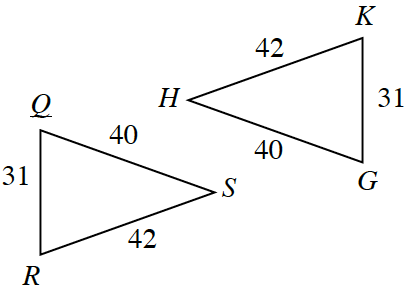Home > INT2 > Chapter 2 > Lesson 2.1.1 > Problem2-6

2-6.

The diagrams below are not necessarily drawn to scale. For each pair of triangles:

• Determine if the two triangles are congruent.

• If the triangles are congruent, write a congruence statement (such as $ΔPQR≅ΔXYZ$) and give the congruence theorem (such as $\operatorname{SAS}≅$).

• If the triangles are not congruent, or if there is not enough information to determine congruence, write “cannot be determined” and explain why not. Homework Help ✎

A shared side is a congruent side.
AAA does not prove congruency.
Order matters: SAS proves congruence but SSA does not.

1. $\overline{AC}$ is a straight segment: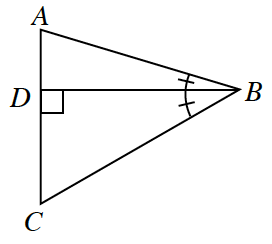1.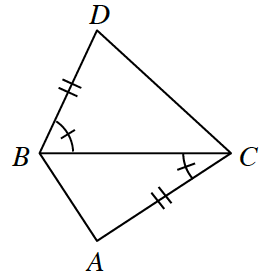1.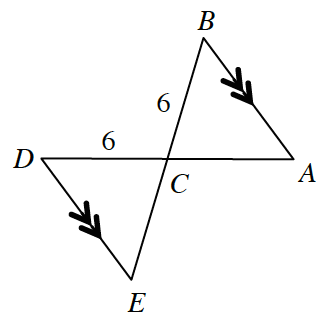1.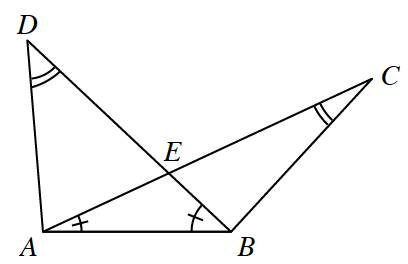1.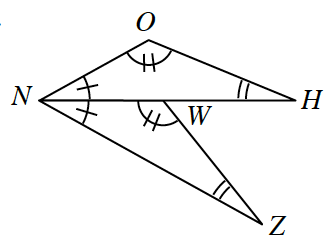1.